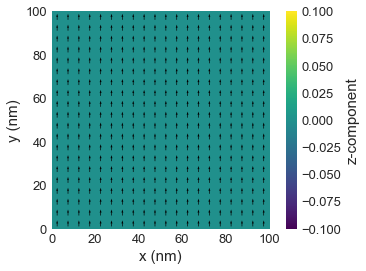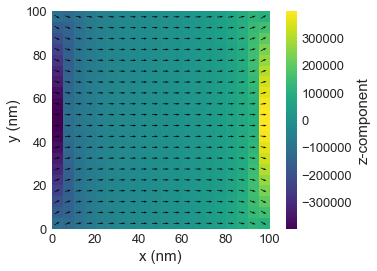# Getting started 00: First Ubermag notebook¶

Interactive online tutorial:The goal of this tutorial is to familiarise users with basics of running Ubermag simulations in Jupyter notebook. The only thing you need to know for this tutorial is how to execute individual cells: this is done by pressing Shift + Return (Return = Enter).

## Simple Ubermag simulation¶

Before we specify and run the simulation, we have to import Ubermag modules we intend to use. Because we are going to run OOMMF simulations, we will import oommfc and for creating finite diference fields, we are going to import discretisedfield.

In :
import oommfc as oc
import discretisedfield as df
import micromagneticmodel as mm

# The following line enables plotting inside the notebook.
%matplotlib inline


### System¶

The object on which different drivers act on in Ubermag is oommfc.System. In order to define the micromagnetic system we intend to simulate, we have to specify:

1. Hamiltonian,
2. Dynamics equation,
3. Magnetisation configuration.
In :
system = mm.System(name='first_ubermag_simulation')


#### Hamiltonian¶

The Hamiltonian for the first Ubermag simulaton is very simple and contains only:

1. exchange,
2. demagnetisation,
3. Zeeman energy terms.

We will apply an external magnetic field in the $x$-direction for the purpose of this demonstration:

In :
A = 1e-12  # exchange energy constant (J/m)
H = (5e6, 0, 0)  # external magnetic field in the x-direction (A/m)
system.energy = mm.Exchange(A=A) + mm.Demag() + mm.Zeeman(H=H)


#### Dynamics equation¶

The dynamics equation contains only precession and damping terms:

In :
gamma0 = 2.211e5  # gyrotropic ratio parameter (m/As)
alpha = 0.2  # Gilbert damping
system.dynamics = mm.Precession(gamma0=gamma0) + mm.Damping(alpha=alpha)


#### Initial magnetisation¶

We initialise the system in positive $y$-direction, i.e. (0, 1, 0), which is different from the equlibrium state we expect for the external Zeeman field applied in $x$ direction:

In :
L = 100e-9  # cubic sample edge length (m)
d = 5e-9  # discretisation cell size (m)
mesh = df.Mesh(p1=(0, 0, 0), p2=(L, L, L), cell=(d, d, d))

Ms = 8e6  # saturation magnetisation (A/m)
system.m = df.Field(mesh, dim=3, value=(0, 1, 0), norm=Ms)


### Inspect the properties of the system¶

We can check the characteristics of the system we defined by asking objects to represent themselves:

In :
mesh.k3d()

In :
system.energy

Out:
$A \left[ (\nabla \mathbf{m}_\text{x})^{2} + (\nabla \mathbf{m}_\text{y})^{2} + (\nabla \mathbf{m}_\text{z})^{2} \right]-\frac{1}{2}\mu_{0}M_\text{s}\mathbf{m} \cdot \mathbf{H}_\text{d}-\mu_{0}M_\text{s} \mathbf{m} \cdot \mathbf{H}$
In :
system.dynamics

Out:
$-\frac{\gamma_{0}}{1 + \alpha^{2}} \mathbf{m} \times \mathbf{H}_\text{eff}-\frac{\gamma_{0} \alpha}{1 + \alpha^{2}} \mathbf{m} \times (\mathbf{m} \times \mathbf{H}_\text{eff})$

We can also visualise the current magnetisation field (using MatPlotLib=mpl):

In :
system.m.plane('z').mpl()And we can visualise the same data using k3d_vectors - this is more suitable for interactive exploration:

In :
system.m.plane('z').k3d_vector(head_size=20)


### Driving the system¶

After the system object is created, we can minimise its energy (relax it) using the Minimisation Driver (MinDriver).

In :
md = oc.MinDriver()
md.drive(system)

Running OOMMF (ExeOOMMFRunner) [2020/06/12 00:33]... (1.8 s)


The system is now relaxed, and we can plot its slice and compute its average magnetisation.

In :
system.m.plane('z').mpl()In :
system.m.plane('z').k3d_vector(color_field=system.m.z, head_size=20)

In :
system.m.average

Out:
(7850881.329363451, -0.00035205061599845067, -2.2642780095338822e-11)

We can see that the magnetisation is aligned along the $x$-direction, as expected having in mind we applied the external magnetic field in that direction.